# Harmonic repulsion potential

The Harmonic repulsion potential, designed for studies of polymer micelles, dendrimers, and colloidal particles, is given by ( Eq. 2):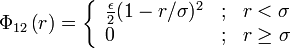$\Phi_{12}\left( r \right) = \left\{ \begin{array}{lll} \frac{\epsilon}{2} (1-r/\sigma)^{2} & ; & r < \sigma \\ 0 & ; & r \geq \sigma \end{array} \right.$

where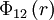$\Phi_{12}\left( r \right)$ is the intermolecular pair potential,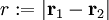$r := |\mathbf{r}_1 - \mathbf{r}_2|$ is the distance between site 1 and site 2.$\sigma$ represents a length scale and$\epsilon$ an energy. For example, for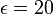$\epsilon=20$ the potential looks like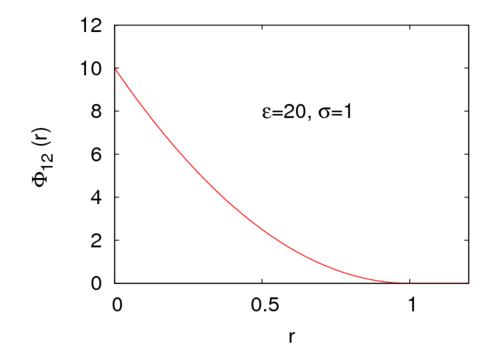## Phase diagram

The phase diagram in the pressure-temperature plane has been calculated by Zhu and Lu .